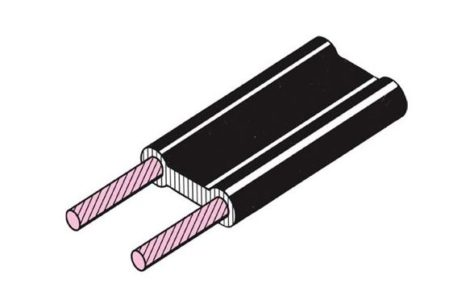Home / Instrumentation / Wire Gauge Sizes | Circular mils

# Wire Gauge Sizes | Circular mils

Want create site? Find Free WordPress Themes and plugins.

In order to compare the resistance and conductor sizes with each other, we need to establish a convenient unit. This unit is the mil-foot (mil-ft). A conductor will possess this unit size if having one mil (0.001 inches) diameter and a length of one foot.

The standardized unit for wire cross-sectional area is circular mil. Since the round conductor’s diameter is generally a small fraction of an inch, it is quite handy to demonstrate them in mils, which is equivalent to 1/1000 of an inch, for avoiding the use of decimals.

For instance, the conductor diameter can be stated as 25 mils instead of 0.025 in. A circular mil, in fact, is the area of a circle whose diameter is one mil, as depicted in figure 1.Fig.1: Circular Mil

The area of a round conductor in circular mils is acquired by squaring up the diameter which is calculated in mils. This convention is an engineering agreement which is in practice for last many decades and is not quite associated with the circle area being equivalent to $\pi {{r}^{2}}$.

Example

What is the circular mil area of a round conductor with a diameter of 25 mils?

Solution

$A={{d}^{2}}={{25}^{2}}=635\text{ c}\text{.mils}$

A circular mil-foot, as demonstrated in figure 2, is, in reality, a volume unit. It holds a one circular mil cross-sectional area and a length of one foot. Since it is conceived a unit conductor, the circular mil-foot is very valuable in order to make comparisons between conductors formed of dissimilar metallic elements. For instance, a resistivity comparison of different conductors’ types can be made by finding out the resistance of a circular mil-foot of each conductor.Fig.2: Circular Mil-Foot

## Specific Resistance or Resistivity

The resistance of a conductor expressed in ohms per unit length per unit area, that is, per circular mil-foot.

Specific Resistance can be described as the resistance (calculated in ohms) offered by a unit volume (which is equivalent to the circular mil-foot) of a substance (material) to the electrical current flow. A substance that possesses higher resistivity will offer low conductivity, and vice versa. For instance, the cooper specific resistance is $10.4\text{ }{}^{\Omega }/{}_{mil-ft}$ . Put differently, a copper conductor of the cross-sectional area and a length of 1 foot possesses 10.4 Ω resistance.

A list of specific resistivities of several different types of materials is given in table 1. The values indicated are based on 20 oC.

MaterialResistivity
Silver9.56
Copper10.4
Gold14
Aluminum17
Tungsten34
Brass42
Iron61
Nichrome675

Table 1: Specific Resistivities (${}^{\Omega }/{}_{c.mil-ft}$  at 20 oC)

## Calculation of Resistance

The relationship of specific resistance, length, and cross-sectional area is given by the following equation:

$R=\rho \frac{L}{A}$

Where

ρ= specific resistance

L= length in feet

A= cross-section area in circular mils

The following example illustrates the use of this formula.

Example

Calculate the resistance of a piece of copper wire at 20 oC if it is 25 ft long and 40 mils in diameter.

Solution

$A={{d}^{2}}={{40}^{2}}=1600\text{ c}\text{.mils}$

Substitute in the above-mentioned formula, we come up with

$R=10.4*\frac{25}{1600}=0.163\text{ }\Omega$

## American Wire Gauge

The system of notation for measuring the size of conductors or wires.

Wires are manufactured in sizes numbered according to the American wire gage (AWG). Some of these numbers appear in Table 1. Notice that the wire diameters become smaller as the gage numbers increase. In typical applications, where the current is mill amperes, a #22 number wire would be used. By comparison, a #14 wire is customarily used in residential-lightning circuits and #12 for wall plugs. When any conductor is selected, consideration must be given to the maximum current it can safely carry and the voltage its insulation can stand without breakdown.

Gage numberDiameter (mils)Circular mil areaOhms per 1000 ft
03651330000.0795
03251060000.1
1289837000.126
2258664000.159
3229526000.201
4204417000.253
5182331000.319
6162263000.403
7144208000.508
8128165000.641
9114131000.808
10102104001.02
119182301.28
128165301.62
137251802.04
146441102.58
155732603.25
165125804.09
174520505.16
184016206.51
193612908.21
2032102010.4
2128.581013.1
2225.364216.5
2322.650920.8
2420.140426.2
2517.932033
2615.925441.6
2714.220252.5
2812.616066.2
2911.312783.4
3010101105
318.979.7133
32863.2167
337.150.1211
346.339.8266
355.631.5335
36525423

Table.2: American Wire Gauge (AWG) Wire Sizes

Copper is most frequently used for wire conductors because it has a low resistance per unit length, is less expensive than silver or gold, and is easily solderable. The copper is usually tinned (covered with a thin coating of solder) and may be solid or stranded.

Twin-lead transmission line

A type of transmission line comprised of two parallel conductors covered by a solid insulation.

Coaxial cable

A transmission line in which one conductor is concentric to another and separated by a continuous solid dielectric spacer.

Many electric cables are used in industry to interconnect components. Cables consist of two or more conductors within a common covering. Figure 1 shows a typical 300 Ω twin-lead transmission line, or cable, such as used in TV to connect the antenna to the receiver.Fig.3: Twin-Lead Transmission Line

The cable shown in figure 2 is a coaxial cable, which is used extensively for conducting high-frequency currents and consists of an inner conductor surrounded by polyethylene or other highly resistive insulation. Over the insulation is a flexible, tinned copper braid, which is in turn enclosed in a vinyl jacket. The inner conductor and braid constitute the two leads.Fig.4: Coaxial Cable

## Aluminum Conductors

Although aluminum has only about 60 % of the conductivity of copper, it is much lighter in weight than copper and is now frequently used by the electrical power companies. Because aluminum conductors are not easily soldered, lugs, or terminals, are generally fastened to them by special tools.

Did you find apk for android? You can find new Free Android Games and apps.

### About Ahmad FaizanMr. Ahmed Faizan Sheikh, M.Sc. (USA), Research Fellow (USA), a member of IEEE & CIGRE, is a Fulbright Alumnus and earned his Master’s Degree in Electrical and Power Engineering from Kansas State University, USA.